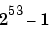MATLAB Function Referencebitmax

Return maximum double-precision floating-point integer

Syntax

• ```bitmax
```

Description

```bitmax ``` returns the maximum unsigned double-precision floating-point integer for your computer. It is the value when all bits are set, namely the value.

 Note    Instead of integer-valued double-precision variables, use unsigned integers for bit manipulations and replace `bitmax` with `intmax`.

Examples

Display in different formats the largest floating point integer and the largest 32 bit unsigned integer:

• ```format long e
bitmax
ans =
9.007199254740991e+015

intmax('uint32')
ans =
4294967295

format hex
bitmax
ans =
433fffffffffffff

intmax('uint32')
ans =
ffffffff
```

In the second bitmax statement, the last 13 hex digits of `bitmax` are `f`, corresponding to 52 1's (all 1's) in the mantissa of the binary representation. The first 3 hex digits correspond to the sign bit 0 and the 11 bit biased exponent `10000110011` in binary (`1075` in decimal), and the actual exponent is (`1075-1023`) = `52`. Thus the binary value of `bitmax` is `1.111...111 x 2^52` with 52 trailing 1's, or `2^53-1`.

See Also

`bitand`, `bitcmp`, `bitget`, `bitor`, `bitset`, `bitshift`, `bitxor`

© 1994-2005 The MathWorks, Inc.